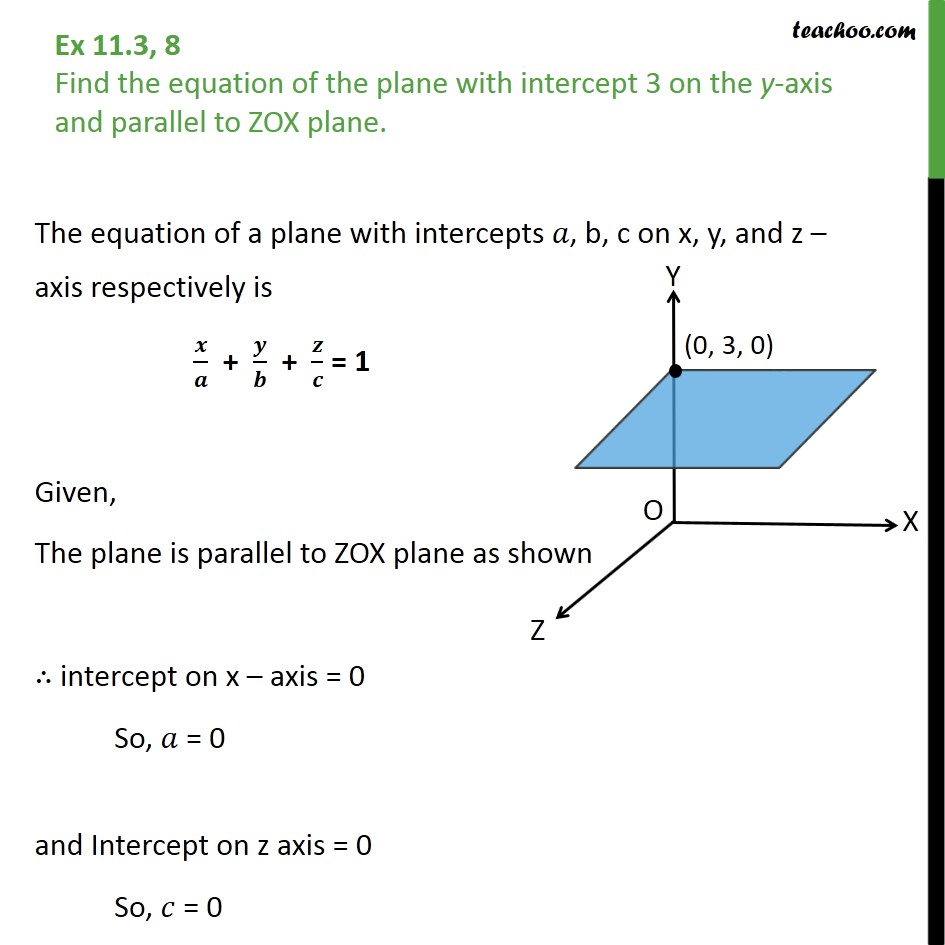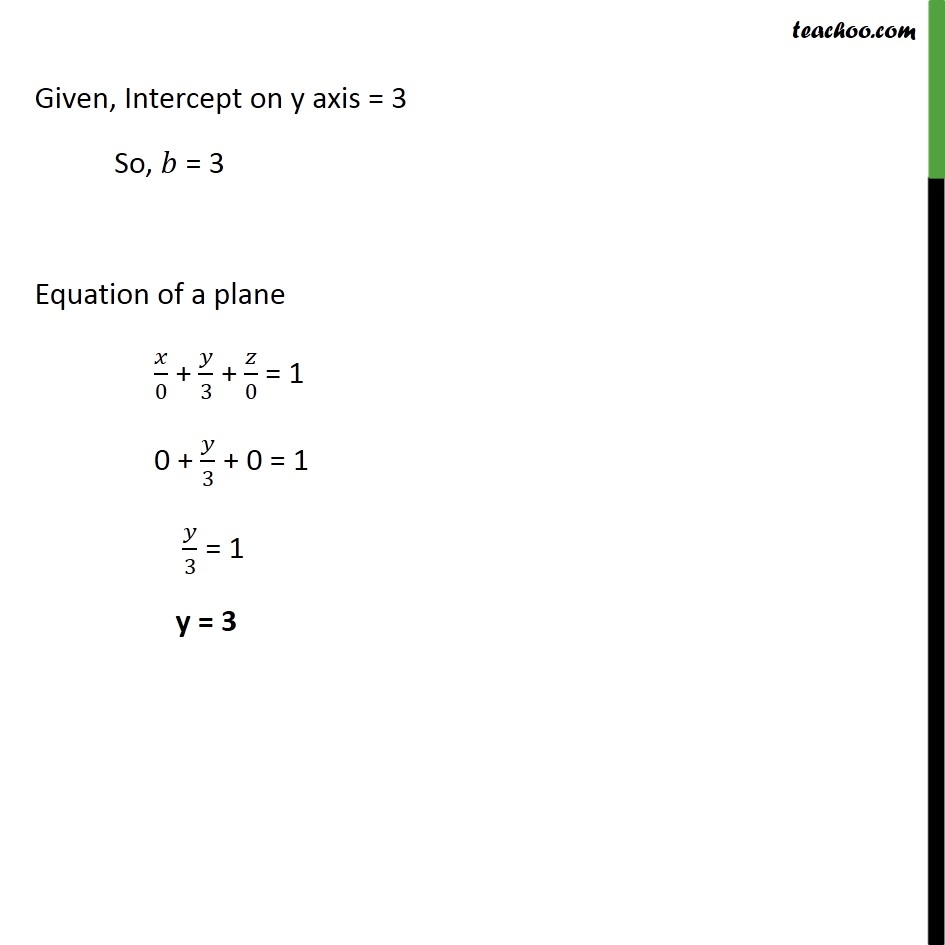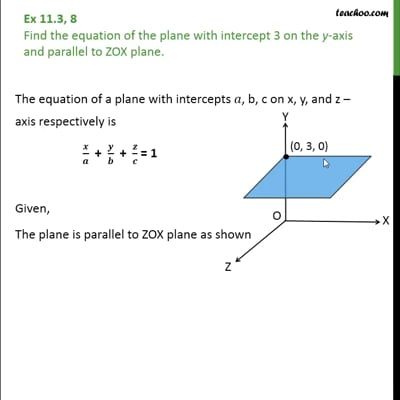Ex 11.3

Chapter 11 Class 12 Three Dimensional Geometry
Serial order wiseThis video is only available for Teachoo black users

Solve all your doubts with Teachoo Black (new monthly pack available now!)

### Transcript

Ex 11.3, 8 Find the equation of the plane with intercept 3 on the y-axis and parallel to ZOX plane. The equation of a plane with intercepts 𝑎, b, c on x, y, and z – axis respectively is 𝒙﷮𝒂﷯ + 𝒚﷮𝒃﷯ + 𝒛﷮𝒄﷯ = 1 Given, The plane is parallel to ZOX plane as shown ∴ intercept on x – axis = 0 So, 𝑎 = 0 and Intercept on z axis = 0 So, 𝑐 = 0 Given, Intercept on y axis = 3 So, 𝑏 = 3 Equation of a plane 𝑥﷮0﷯ + 𝑦﷮3﷯ + 𝑧﷮0﷯ = 1 0 + 𝑦﷮3﷯ + 0 = 1 𝑦﷮3﷯ = 1 y = 3